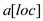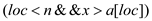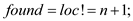# Quiz 12: Sorting

Computing

Program plan: Here in the above, the function "Linear_search" are declaring with the following the arguments: • Declare the required variables. • Include the inbuilt function as "assert(n • Include "while" statement to check the "a[loc]" is not equal to "x" • Increment the location as "loc++". • Assign found as the loc is not equal to the number of array. • Then checking the Boolean expression. o Display the position of the element. • else o Then return the location. • Define the main program o Declares the required variables o Display to get the values o Get the elements using the loop o Calling the function • Then return the value zero Driver Program: / ********************************************************** * Driver Program to test the improved "linearSearch()" * * function * ********************************************************** / / / include the required header files #include #include / / define the capacity size #define capacity 20 using namespace std; / / include Linear_search function with the argument int Linear_search(int a[], int x, int n, bool found, int loc) { / / initials the i int i=n; / / check the size of the array list assert(n / / assign the item into an array a[i] = x; / / set the loc equal to zero loc = 0; / * define a loop condition to check the element in the array * / while (a[loc] != x) / / make increment of loc loc++; / * assign found as the loc is not equal to the number of array * / found = loc != n + 1; / / checking the boolean expression if (found == true) / / display the position of the element cout "\nElement is found at position " loc; return loc; } / / define the main program int main() { / / declares the required variables int a[capacity], n, x, loc = 0; bool found = false; / / display to get the values cout "How many elements?"; cin n; cout "\nEnter elements of the array\n"; / / get the elements using the loop for (int i = 0;i cin a[i]; cout "\nEnter element to search:"; cin x; / / calling the function Linear_search(a, x, n, found, loc); cout endl; / / pause the screen system("pause"); / / return 0 return 0; } Sample Output: How many elements? 3 Enter elements of the array 3 6 7 Enter element to search: 8 Element is found at position 3

Program plan: Here in the above, the function "Linear_search" are declaring with the following the arguments: • Declare the required variables. • Include "while" statement to check the loc greater than n and "x" is greater then, return increment "loc". • Then, assign the "found" as. • Include "if" to check "found" is TRUE. • Then, "else" "found" is FALSE. • Define the main program o Declares the required variables o Display to get the values o Get the elements using the loop o Calling the function o Then return zero Driver Program: / ********************************************************** * Driver Program to test the "linearSearch ()"function for* * ordered list * ********************************************************** / #include #include / / define the capacity size #define capacity 20 using namespace std; / / include Linear_search function with the argument int Linear_search (int a[], int x, int n, bool found, int loc) { / / initials the loc to zero loc = 0; / / include while condition to check on loop while (loc n x a[loc]) / / make increment loc++; / / set the found value found = (loc n a[loc] == x); / / check the condition if (found == true) cout "Element is Found"; else cout "Element not found"; return found; } / / define the main program int main() { / / declares the required variables int a[capacity], n, x, loc = 0,i,j,temp; bool found = false; / / display to get the values cout "How many elements?"; cin n; cout "\nEnter elements of the array\n"; / / get the elements using the loop for (int i = 0;i cin a[i]; cout "\n Enter element to search:"; cin x; / / Sort the elements for (i = 0; i n; ++i) { for (j = i + 1; j n; ++j) { / / Compare the elements and swap if (a[i] a[j]) { temp = a[i]; a[i] = a[j]; a[j] = temp; } } } / / calling the function Linear_search(a, x, n, found, loc); cout endl; / / pause the screen system("pause"); / / return 0 return 0; } Sample Output: How many elements? 5 Enter elements of the array 3 6 9 4 2 Enter element to search: 4 Element is found.

Program plan: Here in the above, the function "Linear_search" are declaring with the following the arguments: • Declare the required variables. • Include "if" statement to check the loc is equal to n, return false. • Then, "else if" to check "a[loc]" is equal to "x", return• Then "else" make recursion for the function through itself. • Define the main program o Declares the required variables o Display to get the values o Get the elements using the loop o Calling the function o Display the position of the element o Return the location Program: / ********************************************************** * Driver Program to test the recursive "linearSearch()" * * function * ********************************************************** / / / include the required header files #include #include / / define the capacity size #define capacity 20 using namespace std; / / include Linear_search function with the argument int Linear_search(int a[], int x, int n, bool found, int loc) { / / initials the i int i = n; / / assign the item into an array a[i] = x; / / check the loc equal to n if (loc == n) return found = false; / / else if check the array element equal to x else if (a[loc] == x) return found = loc != n + 1; / / then       else / / performed recursion function return Linear_search(a, x, n, found, loc=loc+1); } / / define the main program int main() { / / declares the required variables int a[capacity], n, x, loc = 0; bool found = false,flag; / / display to get the values cout "How many elements?"; cin n; cout "\nEnter elements of the array\n"; / / get the elements using the loop for (int i = 0;i cin a[i]; cout "\nEnter element to search:"; cin x; / / calling the function flag = Linear_search(a, x, n, found, loc); if (flag == true) / / display the position of the element           cout "\nElement is found "; else cout "\nElement not found"; / / return the location system("pause"); return 0; } Sample Output: How many elements? 5 Enter elements of the array 3 6 9 5 2 Enter element to search: 9 Element is found.